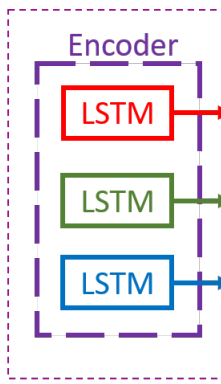Batch size for LSTM

I am working on an encoder that uses LSTMdef init_hidden(self, batch):
'''
used to initialize the encoder (LSTMs) with number of layers, batch_size and hidden layer dimensions
:param batch:  batch size
:return:
'''
return (
torch.zeros(self.num_layers, batch, self.h_dim).cuda(),
torch.zeros(self.num_layers, batch, self.h_dim).cuda()
)

this is the code to initialize the LSTM, what does the batch_size represent for LSTM ? is the number of LSTMs used in the encoder ? . It is from social gan algorithm.

Can someone please explain what it means?

The batch size has the same meaning as in the traditional feed forward models.
It stands for the number of sequences you want to process at the same time.

So your input should be of size (sequence_length, batch_size, number_of_features).

Hi thank you for the reply yea I already know that but I read somewhere that batch_size produces batch_size number of sequences for LSTM … so that’s what I wanted to know if that is true or not ?

If you are talking about the output of a LSTM with that hidden size: the final hidden state is composed by batch_size number of sequences representing the hidden state at each time step, while the output is composed by batch_size vectors representing the hidden state at the last time step.

Hi, thank you for your reply so does it mean the number of batch_size generates that many LSTMs in the image ? for example if the batch size is 3 the there would be 3 LSTMs with their own hidden states respectively?

No, there is only 1 LSTM that produces in output batch_size sequences. It is more or less the same process that occurs in a feedforward model, when you obtain batch_size predictions with just one output layer.

Take a look at the official docs for the LSTM to understand the shape of input and output of the model. In particular the input and output section.

Ah great thank you for the information, I will take a look at the official docs .

Hi,
I am a pretty beginner of DL and Pytorch, and now working on constructing LSTM for training some sequence data.
I am wondering that for example if we have 500 sequence length of data with 20 features. And I want to split them into a batch size of 10. Then when we input the data should we have data with a shape of (500, 10, 20), which has the same 500 sequence and 20 features in each of the batches? Or we should have data with a shape of (50, 10, 20) which 500 sequence data is divided by 10 and they have different data in each batch?

Thank you very much.